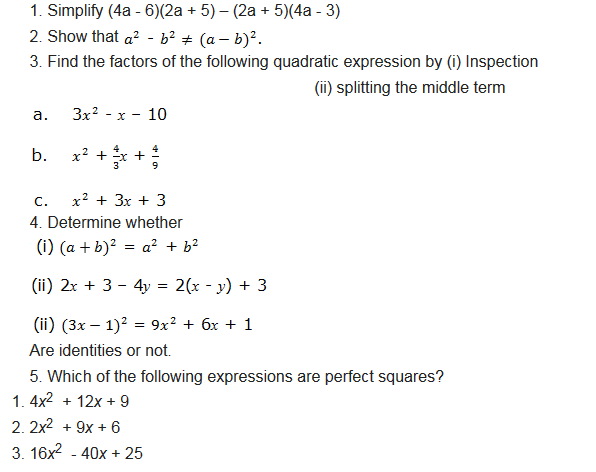# FORM TWO MATHEMATICS STUDY NOTES TOPIC 1-2.

2
737Exponents
Exponents
tell how many times to use a number itself in multiplication. There are
different laws that guides in calculations involving exponents. In this
chapter we are going to see how these laws are used.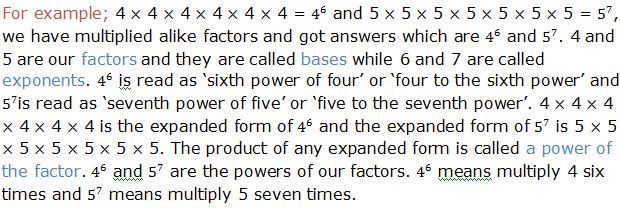Indication of power, base and exponent is done as follows: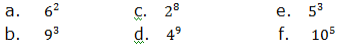Solution: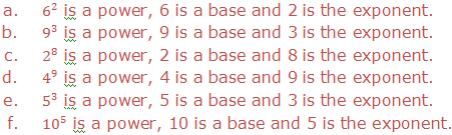To write the expanded form of the following powers: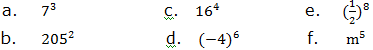Solution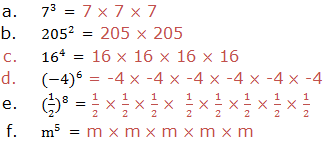To write each of the following in power form: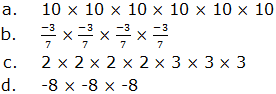Soln.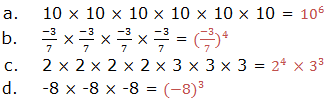The Laws of Exponents
List the laws of exponents
First law:Multiplication of positive integral exponent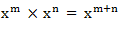Second law: Division of positive integral exponent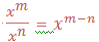Third law: Zero exponents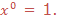Fourth law: Negative integral exponents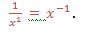Verification of the Laws of Exponents
Verify the laws of exponents
First law: Multiplication of positive integral exponent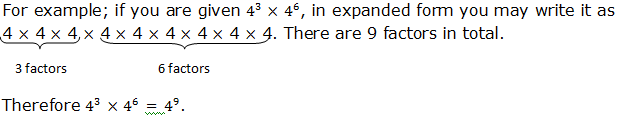Generally,
when we multiply powers having the same base, we add their exponents.
If x is any base and m and n are the exponents, therefore:Example 1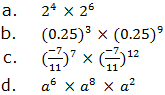SolutionIf you are to write the expression using the single exponent, for example,(63)4.The expression can be written in expanded form as: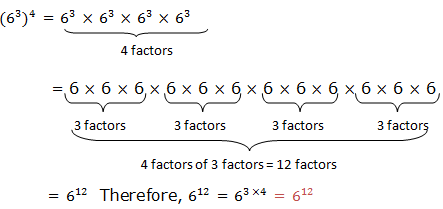Generally if a and b are real numbers and n is any integer,Example 2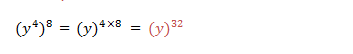Example 3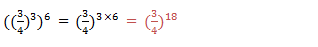Example 4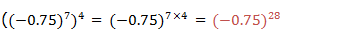Generally, (xm)n = X(mxn)
Example 5
Rewrite the following expressions under a single exponent for those with identical exponents: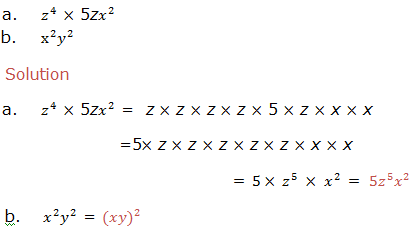Second law: Division of positive integral exponent
Example 6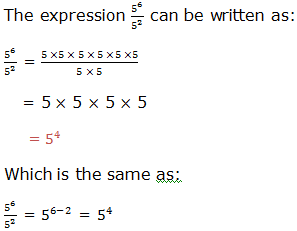Example 7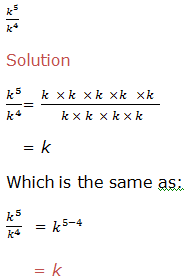Therefore,
to divide powers of the same base we subtract their exponents (subtract
the exponent of the divisor from the exponent of the dividend). That
is,where x is a real number and x ≠ 0, m and n are integers. m is the exponent of the dividend and n is the exponent of the divisor.
Example 8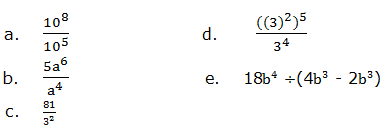solution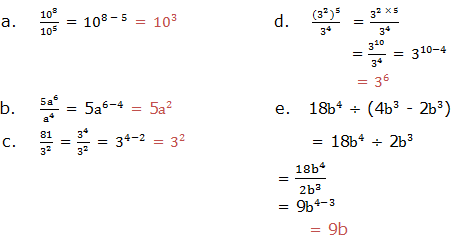Third law: Zero exponents
Example 9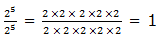This is the same as: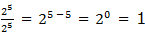If a ≠ 0, then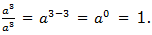Which is the same as: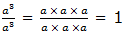Therefore if x is any real number not equal to zero, then X0 = 1,Note that 00is undefined (not defined).
Fourth law: Negative integral exponents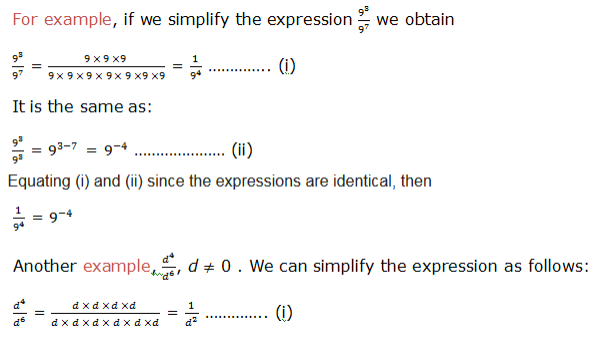Also;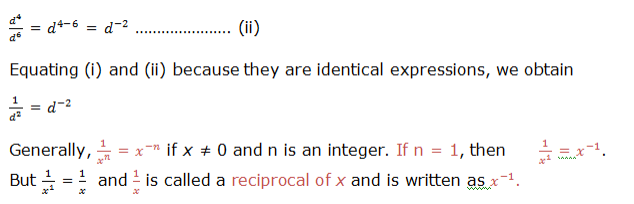Example 10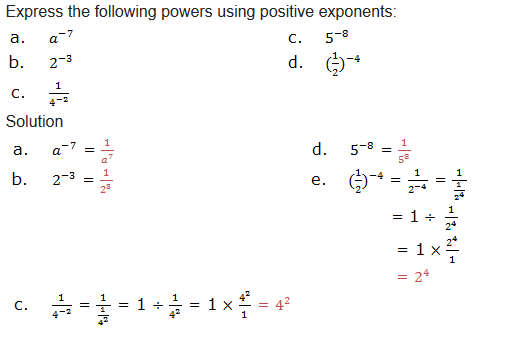Exercise 1
1. Indicate base and exponent in each of the following expressions: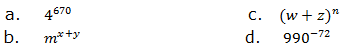2. Write each of the following expressions in expanded form: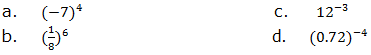3. Write in power form each of the following numbers by choosing the smallest base:
1. 169
2. 81
3. 10,000
4. 625
a. 169 b. 81c. 10 000 d. 625
4. Write each of the following expressions using a single exponent: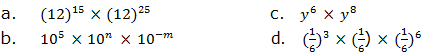5. Simplify the following expressions: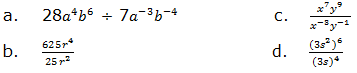6. Solve the following equations: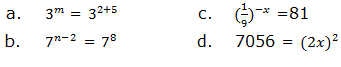7. Express 64 as a power with:
1. Base 4
2. Base 8
3. Base 2
Base 4 Base 8 Base 2
8. Simplify the following expressions and give your answers in either zero or negative integral exponents.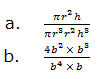9. Give the product in each of the following: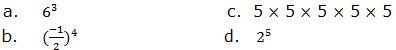10. Write the reciprocal of the following numbers: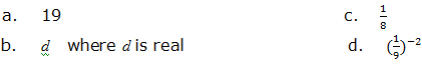Laws of Exponents in Computations
Apply laws of exponents in computations
Example 11SolutionRe-arranging Letters so that One Letter is the Subject of the Formula
Re-arrange letters so that one letter is the subject of the formula
A formula is a rule which is used to calculate one quantity when other quantities are given. Examples of formulas are: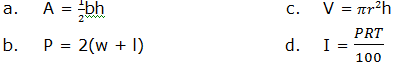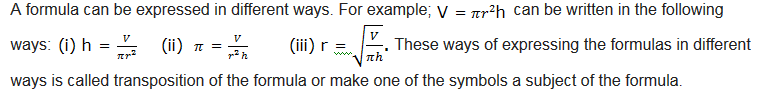Example 14
From the following formulas, make the indicated symbol a subject of the formula: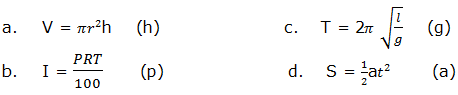Solution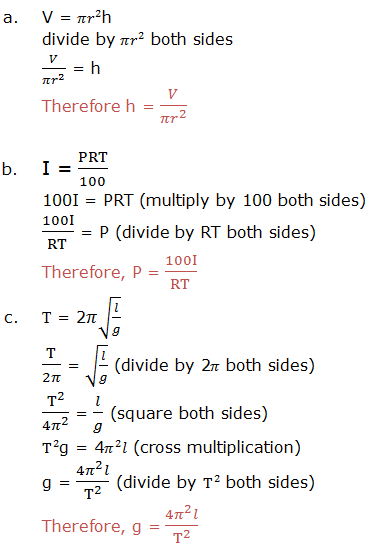Transposing a Formulae with Square Roots and Square
Transpose a formulae with square roots and square
Make the indicated symbol a subject of the formula: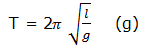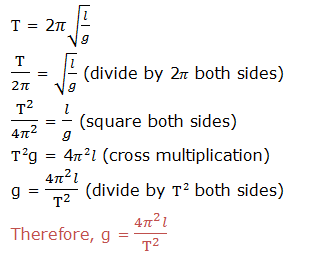Exercise 3
1. Change the following formulas by making the given letter as the subject of the formula.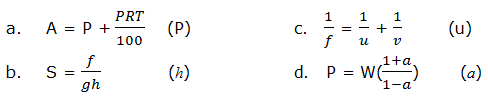2. Use mathematical tables to find square root of each of the following: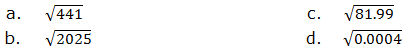When we play games with computers we play by running, jumping and or
finding secret things. Well, with Algebra we play with letters, numbers
and symbols. And we also get to find secret things. Once we learn some
of the ‘tricks’ it becomes a fun challenge to work with our skills in
solving each puzzle. So, Algebra is all about solving puzzles. In this
chapter we are going to learn some of the skills that help in solving
mathematics puzzles.
The Binary Operations
Describe the binary operations
When
two numbers are combined according to the instructions given and
produce one number we say that they are binary operations. For example,
when we add 4 to 6 we get 10, or when we multiply 4 by 3 we get 12. We
see that addition of two numbers lead to one number and multiplication
of two numbers produce one number. This is binary operation. The
instructions can be given either by symbols like X,*,∇and so on or by
words.
Performing Binary Operations
Perform binary operations
Example 1
Evaluate: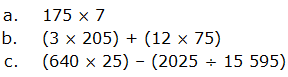solution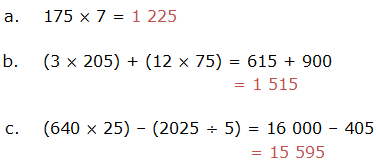Example 2
Find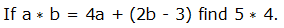solution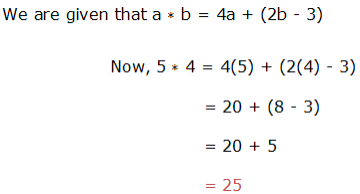Example 3
Solve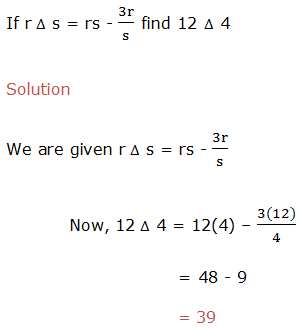Example 4
evaluate,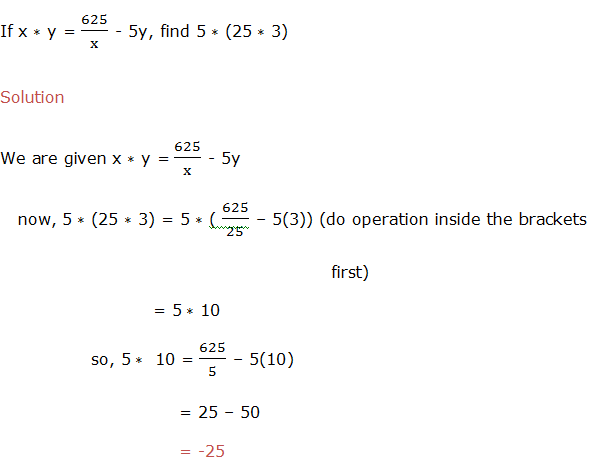Example 5
Calculate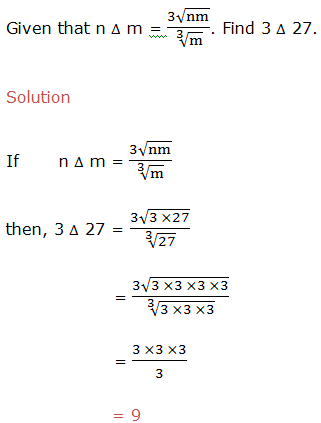Linear Expressions
Factorize linear expressions
The
operation of resolving a quantity into factors, when we expand
expressions, is done by removing the brackets. The reverse operation is
Factorizing and it is done by adding brackets.
Example 11
Factorize the expression 5a+5b.
Solution
In
factorization of 5a+5b, we have to find out a common thing in both
terms. We can see that the expression 5a+5b, have got common coefficient
in both terms, that is 5. So factoring it out we get 5(a+b).
Example 12
Factorize 18xyz-24xwz
Solution
Factorizing
18xyz-24xwz, we have to find out highest common factor of both terms.
Then factor it out, the answer will be 9xz(2y-3w).
When
we write the quadratic expression as a product of two factors we say
that we have factorized the expression. We are going to learn two
methods used to factorize quadratic expressions. These methods are
factorization by Splitting the middle term and factorization by
Inspection.
Factorization by splitting the middle term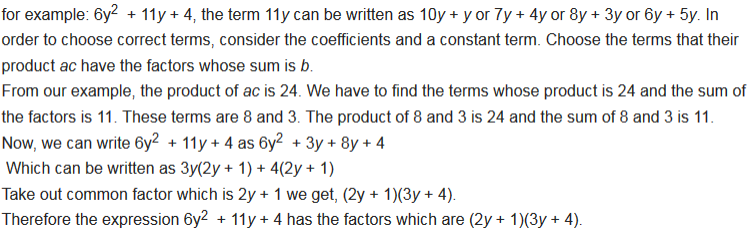Example 13
factorize 3x2 – 2x – 8 by splitting the middle term.
Solution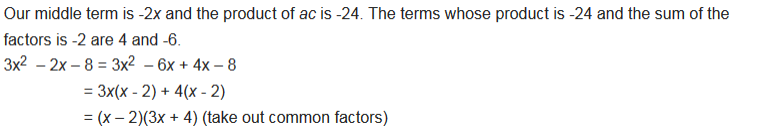Example 14
factorize x2 + 10x + 25 by splitting the middle term.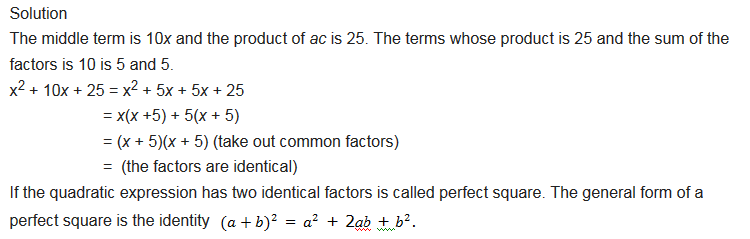Factorization by Inspection
Example 15
factorize x2 + 3x + 2 by inspection.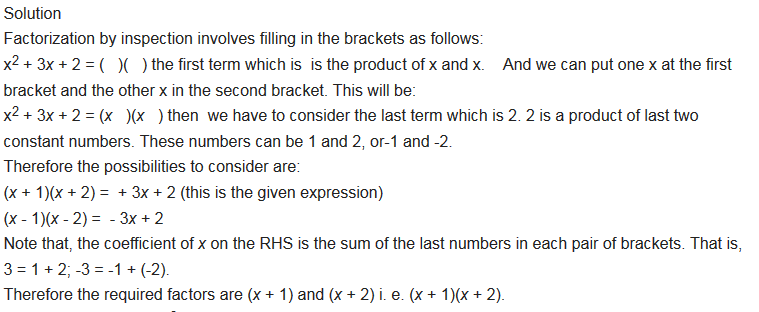Example 16
factorize 4x2 + 5x – 6 by inspection.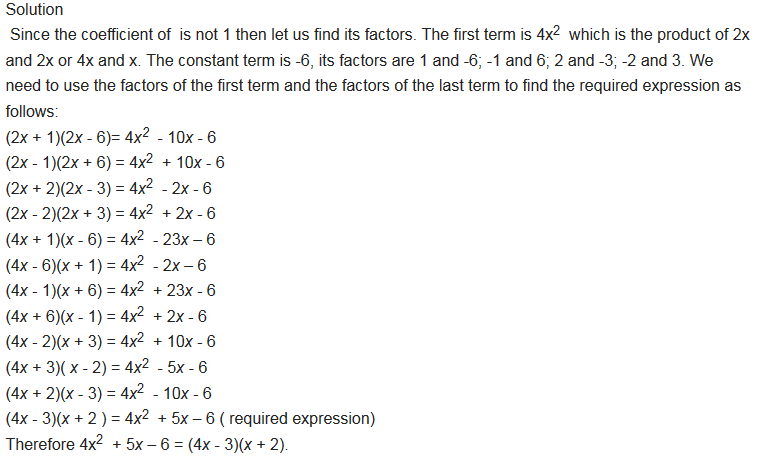Exercise 1
Factorization Exercise;